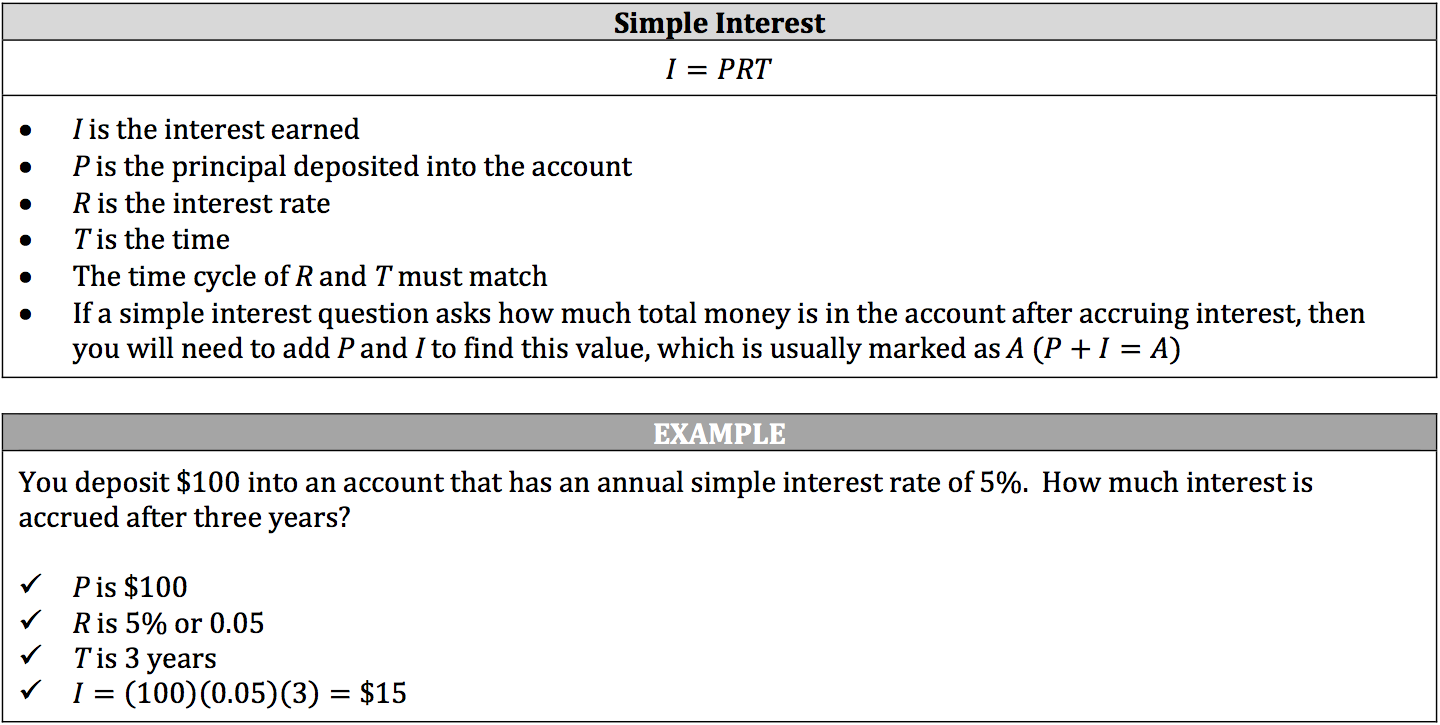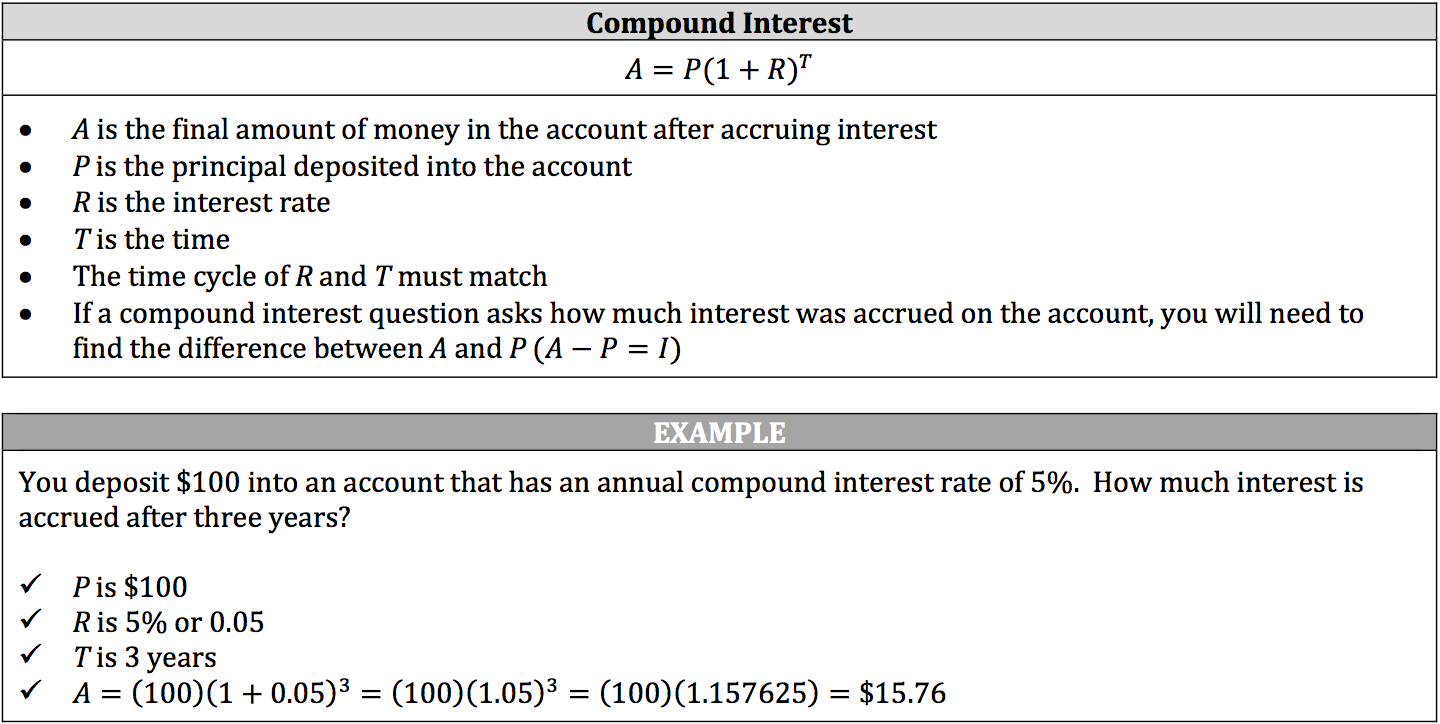Investment word problems involve simple and compound interest. In these problems, an individual deposits a specific amount of money, called the principal, into a bank account that has a particular interest rate. The account accrues interest over a period of time, which is money paid into your account by the bank. Basically, the bank uses your money for investments and pays you for the use of your money (though not very much).

The interest rate is a percentage that is governed by a time cycle, such as an annual rate (the interest is paid once a year). There are two types of interest:

1. Simple interest means that the account accrues interest after a certain amount of time has passed.
2. Compound interest means that the account accrues interest after each time cycle, increasing the principal every time cycle.

The word problem will state whether the account is a simple or compound interest account and will state the time cycle of the interest rate.

### Simple Interest Formula and Example### Compound Interest Formula and Example